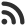Feed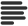Articles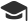Tutorials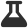Lab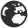CompaniesLeaderboard
 HS Intern at Microsoft May 6, 2020, 3:05 p.m. ⋅ 1166 views

# Modular arithmetic for competitive programming

We often recieve problems in competitive programming and coding contests that require us to calculate mod of something. Though, this is very easy, a lot of beginner fail to implement it.

In this article, I'll cover up all the common concepts that you need to know to about Modular arithmetic.## Quotient Remainder Theorem

Quotient Remainder Theorem says that, if you have any to integers a and b where b is positive, there is always two integers q and r such that a = b x q + r ( where 0 <= r < b )

Let's take  a = 40 and b = 3

We can express it like, 40 = 3 * 13 + 1

So, we'll have q = 13 and r = 1

That's the basic of modular artihmetic. Here r is the mod.

We can perform addition of mods. We'll have to calculate mods of numbers seperately and then add them together and perform mod again of the sum.

``(a + b) mod m = ((a mod m) + (b mod m)) mod m``

Let's take a = 40, b = 3 and m = 5.

So, we want to calculae, (40 + 3) mod 5.

We can do it like following:

(( 40 mod 5 ) + ( 3 mod 5 ) ) mod 5

= ( ( 40 % 5 ) + ( 3 % 5 ) ) % 5

= ( 0 + 3 ) % 5

= 3

That's modular addition. However, same rule is followed for modular substraction as well. You can apply mods seperately on each element and then subtract them, then finally apply mod on output.

## Modular Multiplication

Same distribution approach is followed for modular multiplication as well. We calculate mods of each element seperately, then multiply them, then calcualte the mod of output.

``(a * b) mod m = ((a mod m) * (b mod m)) mod m``

Let's take a = 40, b = 3 and m = 5.

So, we want to calculae, (40 * 3) mod 5.

We can do it like following:

(( 40 mod 5 ) * ( 3 mod 5 ) ) mod 5

= ( ( 40 % 5 ) * ( 3 % 5 ) ) % 5

= ( 0 * 3 ) % 5

= 0

## Modular Inverse

It is not necessary that modular inverse always exists. Modular inverse for any exists only when, gcd(a, m) = 1

In other words, n and m has to be relatively prime.

So, b will be modular inverse of (a mod m) if  (a * b) mod m = 1

Let's take, a = 13 and m = 3

So, to calculate Modular inverse we see, if we plug in 1 as place of b, the mod of (13 * 1) with 3 would be 1. However, 1 is not prime number. It's a co - prime number.

So, next small integer would be 4 for which ( 13  * 4) mod 3 would be 1. So 4 would be modular inverse of (13 mod 3) .

## Modular Division

Previous ways of applying mod to each element and then to final result doesn't work here.

We need to calculate modular inverse first. Since, modular inverse is not always possible to exist, it's alos not always possible to find modular division.

Modular division is given by following way:

``(a / b) mod m = (a * (inverse of b)) mod m``

Inverse of must exist here.

## Modular Exponentiation

To find mod of exponents we can simply calculate the out put of exponent and then apply mod on it.

Suppose we want to calculate (52) mod 3

we can do it like following:

(52) mod 3

=(5 * 5) % 3

= 25 % 3

= 1

Image credits: Image by councilcle from Pixabay

Events

Nov. 28, 2018, 5:30 p.m.

Python from zero to hero

place Delhi

Aug. 13, 2018, 5:30 p.m.

Python from zero to hero

place Bangalore ( HackersFriend office BTM Layout)